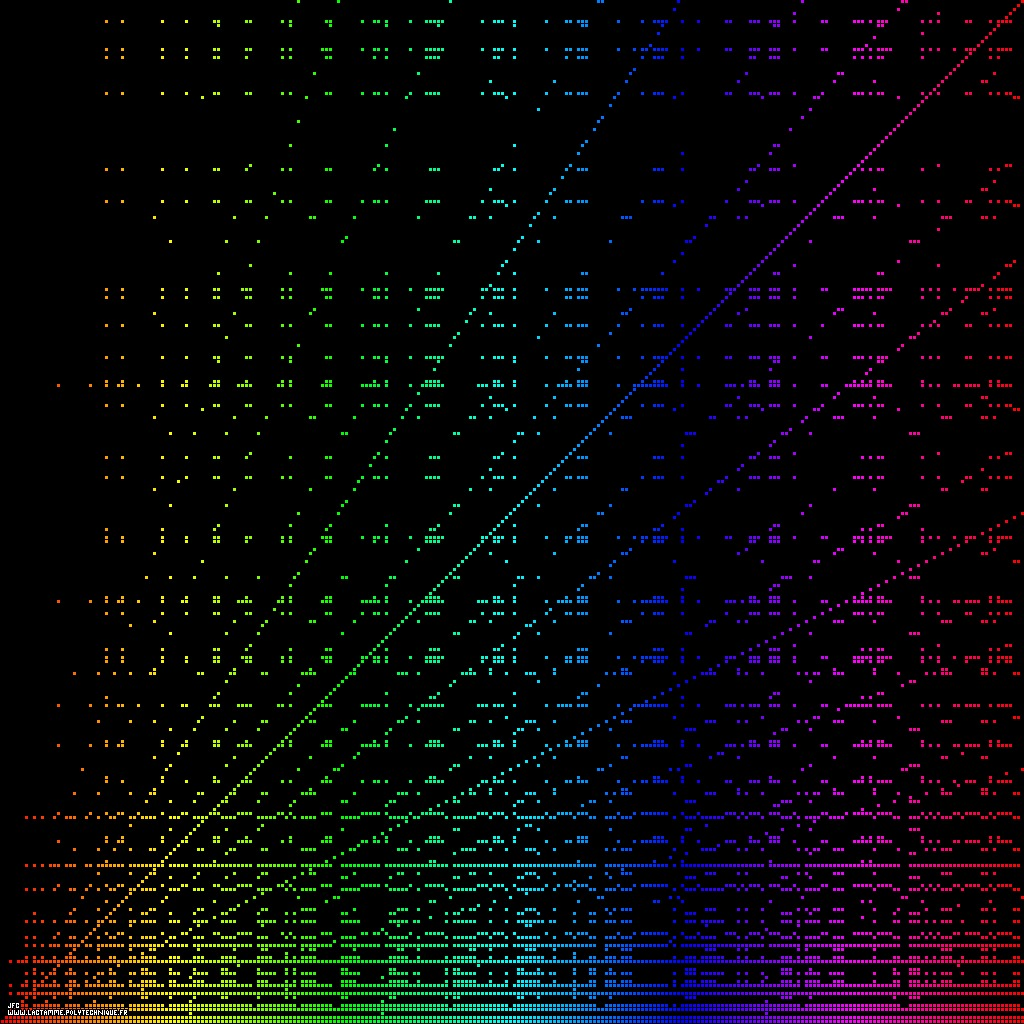The Syracuse conjecture for U(0)={1,2,3,4,...,256} -monodimensional display- [La conjecture de Syracuse pour U(0)={1,2,3,4,...,256} -visualisation monodimensionnelle-].

• The Syracuse sequence is defined as follows:

## ``` U = N (an integer number [un nombre entier]) > 0 0 if U is even [si U est pair] : n n U n U = ---- n+1 2 else [sinon] : U = 3*U + 1 n+1 n ```

• The Syracuse conjecture states that sooner or later the {[[4,] 2,] 1} sequence will appear whatever the starting number N (and then repeats itself obviously ad vitam aeternam). For example with U(0)=7:
```                    U(0)  =  7
U(1)  = 22
U(2)  = 11
U(3)  = 34
U(4)  = 17
U(5)  = 52
U(6)  = 26
U(7)  = 13
U(8)  = 40
U(9)  = 20
U(10) = 10
U(11) =  5
U(12) = 16
U(13) =  8
U(14) =  4
U(15) =  2
U(16) =  1
```

Here are 256 different sequences starting from U(0)=1 to U(0)=256.

• The horizontal and vertical axes represent the integer numbers {1, 2, 3, 4,...}. Each vertical line (with abscissa equals to N) displays the sequence U(n) starting at U(0)=N and the color of each of its points {N,U(n)} is a function of 'N'. For example with N=7, the X=7 vertical line is displayed by means of the 17 following points:
```                    {7,7}  C=7
{7,22} C=7
{7,11} C=7
{7,34} C=7
{7,17} C=7
{7,52} C=7
{7,26} C=7
{7,13} C=7
{7,40} C=7
{7,20} C=7
{7,10} C=7
{7,5}  C=7
{7,16} C=7
{7,8}  C=7
{7,4}  C=7
{7,2}  C=7
{7,1}  C=7
```
where 'C' denotes the color of the points.

See some related visualizations (including this one):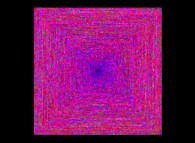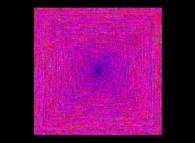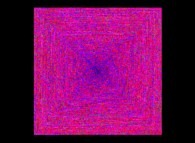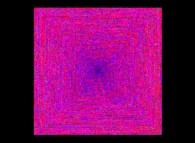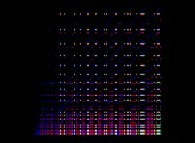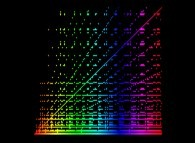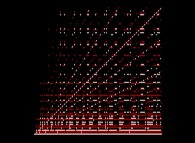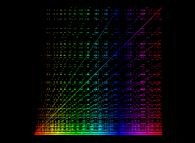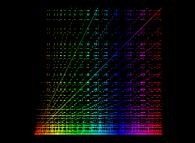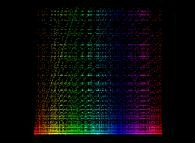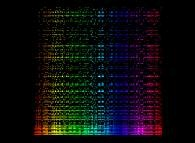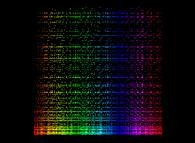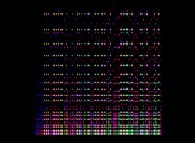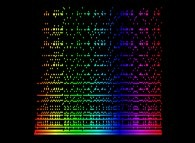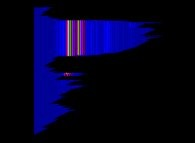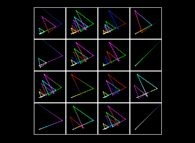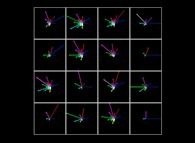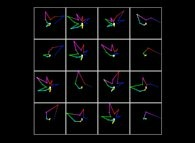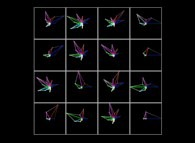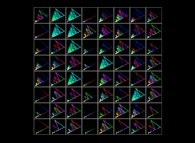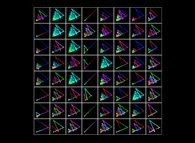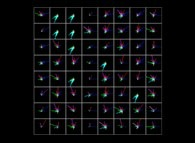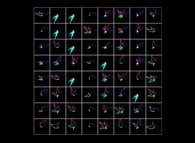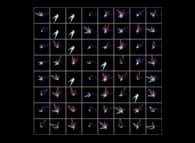(CMAP28 WWW site: this page was created on 01/15/2013 and last updated on 05/06/2020 10:57:56 -CEST-)

[See all related pictures (including this one) [Voir toutes les images associées (incluant celle-ci)]]

[Please visit the related NumberTheory picture gallery [Visitez la galerie d'images NumberTheory associée]]
[Go back to AVirtualSpaceTimeTravelMachine [Retour à AVirtualSpaceTimeTravelMachine]]
[The Y2K bug [Le bug de l'an 2000]]

[Site Map, Help and Search [Plan du Site, Aide et Recherche]]
[Mail [Courrier]]
[About Pictures and Animations [A Propos des Images et des Animations]]